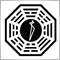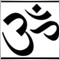# Trouble with my first indicator26

Hi

I'm a newbie with mql4 and when i tried to code my first indicator I could'nt make to draw the arrows on the chart.

An up arrow should be drawn when the volume is more than the extern variable "Vol"; When the close of the bar is higher than the open of the previous, and when the close of the bar is in the upper 75% of that bar.

A Down arrow should be drawn when the volume is more than the extern variable "Vol"; when the close of the bar is lower than the open of the previos, and when the close of the bar is in the lower 25% of that bar.

The indicator works well when I only code the first 2 parameters, but when I include the percentaje parameter it didn't draw any arrow.

I need help undestanding why when i code "((Close[i]-Low[i])/(High[i]-Low[i]))>=0.75"the indicator stops drawing arrows.

Thank you

```//+------------------------------------------------------------------+
//|                                                        Cruxs.mq4 |
//|                                                                  |
//|                                                                  |
//+------------------------------------------------------------------+

#property indicator_chart_window
#property indicator_buffers 2
#property indicator_color1 Aqua
#property indicator_color2 Yellow

//---Indicator Parameters

extern double Vol=4000;

//--- buffers
double UpBar[];
double DownBar[];
//+------------------------------------------------------------------+
//| Custom indicator initialization function                         |
//+------------------------------------------------------------------+
int init()
{
//---- indicators
SetIndexStyle(0,DRAW_ARROW);
SetIndexArrow(0,221);
SetIndexBuffer(0,UpBar);
SetIndexEmptyValue(0,0.0);
SetIndexStyle(1,DRAW_ARROW);
SetIndexArrow(1,222);
SetIndexBuffer(1,DownBar);
SetIndexEmptyValue(1,0.0);
//----
return(0);
}
//+------------------------------------------------------------------+
//| Custom indicator deinitialization function                       |
//+------------------------------------------------------------------+
int deinit()
{
//----

//----
return(0);
}
//+------------------------------------------------------------------+
//| Custom indicator iteration function                              |
//+------------------------------------------------------------------+
int start()
{
int    counted_bars=IndicatorCounted();
int i;
//----

i = Bars-counted_bars-1;
Print (((Close[i]-Low[i])/(High[i]-Low[i])));

while (i>=0)
{
if (Volume[i]>Vol && Close[i]>Open[i+1] && ((Close[i]-Low[i])/(High[i]-Low[i]))>=0.75)
{
UpBar[i]=High[i];
}
if (Volume[i]>Vol && Close[i]<Open[i+1] && ((Close[i]-Low[i])/(High[i]-Low[i]))<=0.25)
{
DownBar[i]=Low[i];
}

i--;
}

//----
return(0);
}
//+------------------------------------------------------------------+

```17419

Cruxs:

I need help undestanding why when i code "((Close[i]-Low[i])/(High[i]-Low[i]))>=0.75"the indicator stops drawing arrows.

Did you check the log or the experts tab ?

What happens if   High[i]-Low[i]   is equal to zero ?26

Hi RaptorUK, thank you for your quick response.

As you can see in the source code I added a "Print" to check the experts log. When High[i]-Low[i] is equal to zero, in the log appears "zero divide" do you thing thats why the indicator stops drawing arrows?17419

Cruxs:

Hi RaptorUK, thank you for your quick response.

As you can see in the source code I added a "Print" to check the experts log. When High[i]-Low[i] is equal to zero, in the log appears "zero divide" do you thing thats why the indicator stops drawing arrows?

Your print is outside of the while loop so it only prints once so is not much of a help . . .  yes a zero divide will stop the Indicator.26

Thank you very much, i'll think how to work this around...17419

Cruxs:

Thank you very much, i'll think how to work this around...

You need to check to see if  High[i]-Low[i]  gives you a zero before you try to divide by it.  If it does equal zero then you could simply use 1 point in it's place . . .  alternatively choose some other default action.5301

Cruxs: Hi RaptorUK, thank you for your quick response. As you can see in the source code I added a "Print" to check the experts log. When High[i]-Low[i]  is equal to zero, in the log appears "zero divide" do you thing thats why the indicator stops drawing arrows?

Yes, Zero Divide will Stop any code. Always look out for Zero_Divide when using /. To solve the problem, check:

```if( High[i]-Low[i] != 0 ){
if (Volume[i]>Vol && Close[i]>Open[i+1] && ((Close[i]-Low[i])/(High[i]-Low[i]))>=0.75){
UpBar[i]=High[i];
}
}```

Additional notes: Start using variable names for stuff like High[i]-Low[i]. Makes it easier to read.

Example: double Hi_minus_Lo=High[i]-Low[i]; __________ if( Hi_minus_Lo != 0 ){}

**Be careful using Volume[i] because you can miss Volumes or Ticks.26

Thank you both for all your help, ubzen solution is much more refine that what i did. I just added a very small number to the expresion: (High[i]-Low[i]+0.00000000000000000001]  Now the indicator works fine for my purposes.